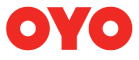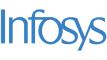New update is available. Click here to update.
Last Updated: 21 Nov, 2020

# Next Permutation

Moderate+18 more companies

## Problem statement#### For example, [2, 1, 3, 4] is lexicographically smaller than [2, 1, 4, 3].

##### Input Format:
``````The first line contains a single integer ‘T’ representing the number of test cases.

The first line of each test case will contain an integer ‘N’ representing the length of the permutation.

The second line contains ‘N’ space-separated integers which are the elements of the permutation.
``````
##### Output Format:
``````For each test case, print the elements of the lexicographically next greater permutation with a single space-separated. If lexicographically next greater permutation doesn’t exist, print the lexicographically smallest permutation.

Output for every test case will be printed in a separate line.
``````
##### Note:
``````You do not need to print anything; It has already been taken care of.
``````
##### Constraints:
``````1 <= T <= 50
1 <= N <= 10000
1 <= P[i] <= N

Time limit: 1 sec
``````## Approaches

### 01 ApproachThe basic idea is to generate all the possible permutations of ‘N’ integers and find the lexicographically next greater permutation.

Steps are as follows:

1. Generate all the possible permutations of ‘N’ integers.
2. Store all the permutations in a 2D array/list.
3. Sort the list of permutations in lexicographically ascending order.
4. Find the index of the input permutation in the sorted list. Let say the index is ‘i’.
• If the given permutation is present at the end of the array/list, no other greater permutation can exist, so print the smallest permutation.
• Otherwise, return the permutation which is present at the (i+1)th index in the list.